# Determining Long Distance Phone Calls, Based on the Number Dialed

To determine long distance phone calls based on the number dialed, we need to use the IF, AND, LEFT, and MID functionsin Microsoft Excel 2010 and 2013.

IF: - Check whether a condition is met and returns one value if True and another value if False.

Syntax of “IF” function =if(logical test,[value_if_true],[value_if_false])

First the formula will perform the logical test and return one output if the value is true and another if false.

For example:Cells A2 and A3 contain the numbers 3 and 5.  If the number is 3 then the formula should display “Yes” otherwise “No”.

The formula is =IF (A1=3,"Yes","No")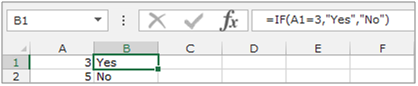LEFT: Returns the specified number of characters starting with the left-most character in the string.

Syntax of “LEFT” function:            =LEFT (text,[num_chars])

Example:Cell A1contains the text “Broncho Billy Anderson”

=LEFT (A1, 7), function will return “Broncho”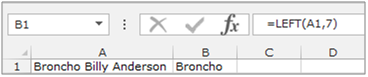MID:Returns a specific number of character(s) from a text string, starting at the position specified based on the number of characters specified.

Syntax of “MID” function:             =MID (text,start_num,num_chars)

Example:Cell A2 contains the text “Broncho Billy Anderson”

=MID (A1, 8, 7), function will return “Billy”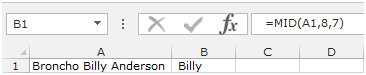AND:- This function is used to check whether all arguments are true and returns TRUE if all arguments are TRUE.  If even one argument is FALSE, it will return FALSE.

The syntax of “AND” function            =AND(logical1,[logical2],….)

For example:Cell A1 contains student name, B1 contains 50, and we need to check the criteria.

• Our criteria is that we need to check if the number is more than 40 and less than 60.
• Write the formula in cell C1
• =AND(B2>40,B2<60), press Enter on the keyboard
• The function will return True.
• If we will change the criteria to less than 40 and greater than 60, the function will return False.
• The value entered in B2 is 90, which does not meet both the criteria (>40, <60), hence it returns FALSE.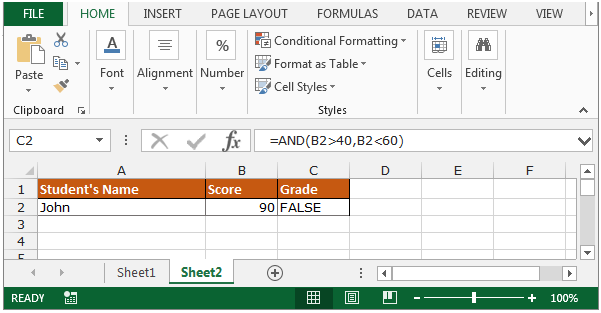Let’s take an example to understand how we can determine long distance phone calls based on the number dialed.

Column A contains a lists of numbers dialed on a particular day.All numbers starting with “1?, except for those starting with “1800? are for long distance calls. We need to analyze each number in column A and determine whether or not it is a long distance call.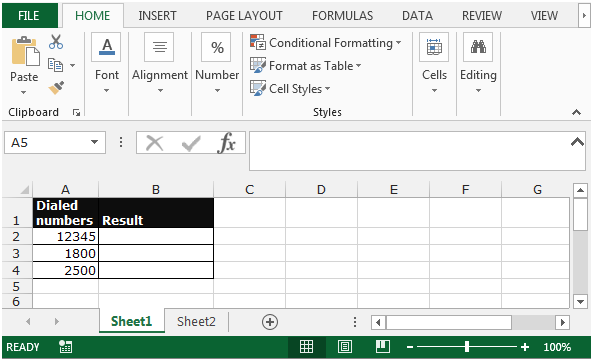Follow the below given steps to determine the long distance phone calls based on the number dialed:-

• Select the cell B2.
• Write the formula=IF(AND(LEFT(A3,1)="1", MID(A3,2,3)<>"800"),"Long Distance", "Not Long Distance")
• Press Enter on your keyboard.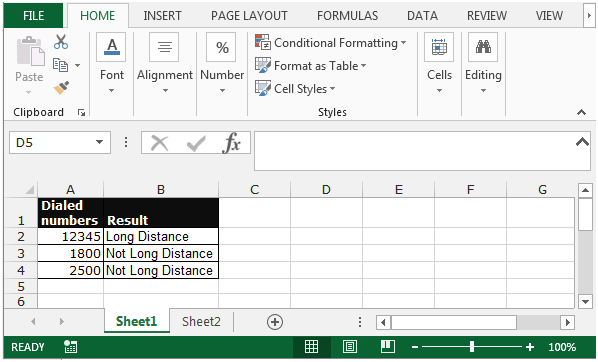• The function will return the output as “long distance”and“not long distance”.

This is the way we candetermine the long distance phone calls, based on the number dialed in Microsoft Excel.

## Users are saying about us...

1.Do Long Distance Relationships Work

I enjoy what you guys are usually up too. This sort of clever work and reporting! Keep up the good works.

Terms and Conditions of use

The applications/code on this site are distributed as is and without warranties or liability. In no event shall the owner of the copyrights, or the authors of the applications/code be liable for any loss of profit, any problems or any damage resulting from the use or evaluation of the applications/code.